# Numerical Study of Mixed Convective Flow of a Couple Stress Fluid in a Vertical Channel with First Order Chemical Reaction and Heat Generation/Absorption

Numerical Study of Mixed Convective Flow of a Couple Stress Fluid in a Vertical Channel with First Order Chemical Reaction and Heat Generation/Absorption

Patil Mallikarjun Ranganatha Vasudeva Murthy Ulavathi Shettar MahabaleshwarGiulio Lorenzini

Department of Studies & Research in Mathematics, Tumkur University, Tumkur 572103, India

Department of Mathematics, Government First Grade College for Women, Hassan 577002, India

University of Parma, Department of Engineering and Architecture, Parco Area delle Scienze 181/A, Parma 43124, Italy

Corresponding Author Email:
ulavathi@gmail.com
Page:
175-182
|
DOI:
https://doi.org/10.18280/mmep.060204
22 January 2019
|
Accepted:
5 April 2019
|
Published:
20 June 2019
| Citation

OPEN ACCESS

Abstract:

The problem of mixed convective flow of a couple stress fluid in a vertical channel in the presence of first order chemical reaction and heat generation/absorption is studied. Two walls of the channel are considered with asymmetric heating conditions along with flux boundary conditions on temperature and concentrations. The governing nonlinear differential equations are solved numerically. The effect of physical parameters like couple stress, heat generation/absorption, chemical reaction, thermal buoyancy and concentration buoyancy are evaluated numerically. Also heat transfer parameters such as Nusselt number, Sherwood number and skin friction factors are analyzed.

Keywords:

mixed convection, couple stress fluid, chemical reaction, vertical channel, numerical method

1. Introduction

Channel flows have been studied substantially due to their increased importance in the various fields of science and engineering. Mixed convection occurs when both free and forced convection have significant effect on heat transfer. In Mixed convection, both buoyancy and pressure forces interact. The common cases are on heating or cooling of channel walls, and at the small velocities of fluid flow that are characteristic of laminar flow, mixed convection is realized. Forced convection (Laminar) may be obtained in capillaries. Mixed convection is normally observed in high-powered-output devices, like Nuclear reactor technology, electronic cooling, etc. In electronic cooling, the components are assembled on the circuit card, an array of which is placed vertically so that forming the vertical channel through this a coolant is passed. Also mixed convection has applications in solar systems, heat exchangers.

In the classification of non–Newotonian fluids, couple stress fluids have been considered distinctly for their high viscosity and polar effects. The noncentral forces of interaction between particles is the reason for the development of polar fluids, which are from the statistical mechanics model. The interparticle couple and interparticle force contribute to the rotation of fluid particles relative to the sourounding particles. The effect of angular momentum like couple stress and symmetric stress tenosors are considered to discriminate the theory of the polar fluids from non – polar fluids. Couple stress fluid study with chemical reaction has enormous applications in the field of biofluid. synthetic lubricants and colloidal fluids. There are attempts by researchers to assume blood as fluid with couple stress.

Umavathi et al.  have studied the flow and heat transfer of two immiscible viscous and couple stress permeable fluids in a vertical channel and showed that the flow can be monitered by selecting varied liquids. Malashetty et al.  have investigated the effect of porous stratum in a couple stress fluid. The blood flow through artery with mild stenosis with the effects of couple stress has been presented by Sinha and Singh . Umavathi  has considered free flow of couple stress fluid which is also electrically conducting in a vertical channel. Umavathi et al.  have investigated the heat transfer of two fluids such as viscous and couple stress fluids in a vertical channel. The poiseuille flow of couple stress fluid with blood flow applications is studied by Valanis et al.  The effect of viscous dissipation on fully developed mixed convection is analyzed for the laminar flow in a parallel plate vertical channel whose walls exchange heat with an external fluid, with third kind boundary conditions has been studied by E. Zanchini . Barletta  pointed out the relevant effects of laminar mixed convection with viscous dissipation in a vertical channel and flow reversal in vertical duct with uniform heat fluxes. The effect of the choice of the reference fluid temperature on the solution of fully developed mixed convection problems in a plane vertical channel has been studied in detail by A Barletta et. al. . An analysis of combined forced and free convection in a vertical parallel plate channel with prescribed wall heat fluxes is investigated by A Barletta .

The increased scope for chemical reaction in chemical and hydrometallurgical industries, the study of heat transfer with chemical reaction has become the need of the hour. There are many transport processes governed by the combined action of buoyancy forces due to both thermal and mass diffusion in the presence of chemical reaction. These are commonly encountered in nuclear safety and combustion systems, solar collectors, metallurgical and chemical engineering. Also the other applications include binary alloys and crystal growth dispersion of dissolved materials of particulate water in flows, drying and dehydration operations in chemical of atomized liquids. Anjalidevi and Kandaswamy  investigated the effect of chemical reaction and heat and mass transfer on laminar flow along a semi infinite horizontal plate. Fully developed laminar mixed convection flow in a vertical channel in the presence of first order chemical reactions has been studied by J Prathap Kumar et al. . An analytical solution of dispersion of solute with first order chemical reaction in a vertical double passage channel filled with porous medium has been analyzed by J. Prathap Kumar et al. , Muthucumaraswamy and Ganeshan  have investigated the impulsive motion of a vertical plate with heat flux/mass, flux/suction and diffusion of chemically reactive species. Seedak  has used the finite element method for studying the effect of chemical reaction, variable viscosity, thermophoresis and heat generation/absorption on a boundary layer hydro magnetic flow with heat and mass transfer over a heat surface. Prathap Kumar et al.  considered the homogeneous and heterogeneous chemical reactions in immiscible fluids. R. Muthucumaraswamy, P. Ganesan , have investigated the first order chemical reaction on flow past an impulsively started vertical plate with uniform heat and mass flux. P Mallikarjun et al.  studied mixed convective fully developed flow in a vertical channel in the presence of thermal radiation and viscous dissipation. B.P. Mallikarjun et al.  have worked on, laminar Magneto Convection flow in a Vertical channel in the presence of heat generation and absorption.

The aim of this study is to take forward the studies in literature on vertical channel with viscous dissipation, heat generation/absorption and first order chemical reaction of couple stress fluids. The effect of first order chemical reaction on mixed convection of couple stress fluids is rare in the literature. So this study is most relevant for the purpose of the chemical industries with heat and mass transfer particularly synthetic colloids and liquid crystals. In this present consideration, the temperature, velocity and concentration profiles are obtained by numerical method based on thermal Grashoff, concentration Grashoff, heat generation/absorption, couple stress and chemical reaction parameters along with Brinkman number, Reynolds number, Nusselt numbers, Sherwood numbers.

2. Mathematical Model

Newtonian, laminar, incompressible couple stress fluid flowing steadily through a parallel plate vertical channel is considered for the present study. The thermal properties like thermal diffusivity, thermal expansion coefficients and dynamic viscosity are taken constant. The Boussinesq approximation and equation of state is applicable. Spatial coordinate system is presented in Figure 1. In axial axis X – axis is considered parallel to the gravitational field, but with opposite direction. The only nonzero component of the velocity field $\vec{q}=U \hat{\imath}+V \hat{\jmath}+W \hat{k}$ is the X – component U.

$\rho=\rho_{0}\left[1-\beta_{T}\left(T-T_{0}\right)-\beta_{C}\left(C-C_{0}\right)\right]$ (1)

$\frac{\partial U}{\partial X}=0$ (2)

Therefore U depends only Y. Hence the momentum balance, energy balance and concentration balance equations for couple stress fluid with no body couple are as follows X – Momentum balance equation

$\beta_{T} g\left(T-T_{0}\right)+\beta_{C} g\left(C-C_{0}\right)-\frac{1}{\rho_{0}} \frac{\partial P}{\partial X}$

$+\frac{\mu}{\rho_{0}} \frac{d^{2} U}{d Y^{2}}-\frac{\eta}{\rho_{0}} \frac{d^{4} U}{d Y^{4}}=0$ (3)

Y – Momentum balance equation\

$\frac{\partial P}{\partial Y}=0$ (4)

where, $P=p+\rho_{0} g X$ is the difference between the pressure and the hydrostatic pressure. Because of the equation (4), P depends only on X therefore equation (3) is expressed as

$\beta_{T} g\left(T-T_{0}\right)+\beta_{C} g\left(C-C_{0}\right)-\frac{1}{\rho_{0}} \frac{d P}{d X}$

$+\frac{\mu}{\rho_{0}} \frac{d^{2} U}{d Y^{2}}-\frac{\eta}{\rho_{0}} \frac{d^{4} U}{d Y^{4}}=0$ (5)

In equation (5) the last term accounts for the effect of couple stress in the fluid.

## f1.pngFigure 1. Schematic diagram

Assuming walls of the channel are isothermal, temperature at left wall $Y=-\frac{L}{2}$ is $T_{1}$, and at the right wall $Y=\frac{L}{2}$, with $T_{2}$ , these thermal boundary conditions are applicable if and only if $\frac{d P}{d X}$ does not depend on X.

Hence $\frac{d P}{d X}=F$ (6)

where, F is a constant.

Therefore differentiating (5) with respect to X we get

$\frac{d T}{d X}=0$ (7)

Hence temperature is dependent on only Y.

Energy balance equation with heat generation/absorption

$\alpha_{1} \frac{d^{2} T}{d Y^{2}}+\frac{v}{C_{p}}\left(\frac{d U}{d Y}\right)^{2} \pm \frac{Q\left(T-T_{0}\right)}{\rho_{0} C_{p}}=0$ (8)

Using equation (5) and (8) we get

$\frac{d^{6} U}{d Y^{6}}=\left(\frac{\mu}{\eta} \mp \frac{Q}{K}\right) \frac{d^{4} U}{d Y^{4}}-\frac{\mu \rho_{0} g \beta_{T}}{K \eta}\left(\frac{d U}{d Y}\right)^{2}$

$-\frac{g \beta_{c} \rho_{0}}{\eta} \frac{d^{2} C}{d Y^{2}} \mp \frac{Q g \beta_{C}}{\alpha_{1} \eta}\left(C-C_{0}\right) \mp \frac{Q F}{K v \rho_{0}}$

$\pm \frac{Q}{K} \frac{d^{2} U}{d Y^{2}}$ (9)

No slip boundary conditions on velocity are assumed

$U\left(-\frac{L}{2}\right)=U\left(\frac{L}{2}\right)=0$ (10)

and boundary conditions on U induced by the boundary conditions on T and by equation (5) and (6) are

$\left.\frac{d^{2} U}{d Y^{2}}\right|_{T_{-}-\frac{L}{2}}=0$, $\left.\frac{d^{2} U}{d Y^{2}}\right|_{T-\frac{L}{2}}=0$

$\left.\frac{d^{4} U}{d Y^{4}}\right|_{x_{-\frac{L}{2}}}=-\frac{F}{\eta}+\frac{\beta_{T} g \rho_{0}\left(T_{1}-T_{0}\right)}{\eta}+\frac{\beta_{C} g \rho_{0}\left(C_{1}-C_{0}\right)}{\eta}$

$\left.\frac{d^{4} U}{d Y^{4}}\right|_{T_{-} \frac{L}{2}}=-\frac{F}{\eta}+\frac{\beta_{T} g \rho_{0}\left(T_{2}-T_{0}\right)}{\eta}+\frac{\beta_{C} g \rho_{0}\left(C_{2}-C_{0}\right)}{\eta}$ (11)

Concentration balance equation

$D \frac{d^{2} C}{d Y^{2}}-K C=0$ (12)

The boundary conditions on concentrations are, at the left wall $Y=-\frac{L}{2}$ is $C_{1}$ and at the right wall $Y=\frac{L}{2}$ is $C_{2}$ with $C_{2} \geq C_{1}$.

Non-dimensional parameters that are used to get dimensionless equations as follows

$u=\frac{U}{U_{0}}$, $\operatorname{Re}=\frac{U_{0} H}{v}$, $y=\frac{Y}{H}$, $\theta=\frac{T-T_{o}}{\Delta T}$, $\phi=\frac{C-C_{o}}{\Delta C}$, $B r=\frac{\mu U_{0}^{2}}{K \Delta T}$, $G r_{T}=\frac{g B_{T} \Delta T H^{3}}{v^{2}}$, $G r_{C}=\frac{g B_{c} \Delta C H^{3}}{v^{2}}$, $\lambda_{T}=\frac{G r_{T}}{\mathrm{Re}}$, $\lambda_{C}=\frac{G r_{C}}{R e}$, $\frac{1}{a^{2}}=\frac{\eta}{\mu H^{2}}$, $\psi=\frac{Q H^{2}}{K}$, $\alpha^{2}=\frac{K H^{2}}{D}$, $R_{C}=\frac{C_{2}-C_{1}}{\Delta C}$, $R_{T}=\frac{T_{2}-T_{1}}{\Delta T}$ (13)

where H = 2L is the hydraulic diameter. Also, the reference velocity $U_{0}$ , temperature $T_{0}$  and concentration $C_{0}$  are given by

$U_{0}=-\frac{F H^{2}}{48 \mu}, T_{0}=\frac{T_{1}+T_{2}}{2}, C_{0}=\frac{C_{1}+C_{2}}{2}$ (14)

The reference temperature difference $\Delta T$ and concentration difference $\Delta C$ is given by

$\Delta T=T_{2}-T_{1}$ if

$T_{1}<T_{2}$ or by $\Delta T=\frac{v^{2}}{C_{p} H^{2}},$ and $\Delta C=C_{2}-C_{1}$ (15)

The values of $R_{T}$ and $R_{C}$ can only be 0 or 1. These values respectively for symmetric and asymmetric heating and concentrations.

The non-dimensional mean velocity $\overline{u}$ bulk temperature $\theta_{b}$ and bulk concentration $\phi_{b}$ are given by

$\overline{u}=2 \int_{-\frac{1}{4}}^{\frac{1}{4}} u d y, \theta_{b}=\frac{2}{\overline{u}} \int_{-\frac{1}{4}}^{\frac{1}{4}} u \theta d y$ and $\phi_{b}=\frac{2}{\overline{u}} \int_{-\frac{1}{4}}^{\frac{1}{4}} u \phi d y$ (16)

From (6) F can be either positive or negative. If F is positive then reference velocity, Reynolds number, thermal buoyancy parameter and concentration buoyancy parameter is negative, hence the flow is downward and vice versa, if F is negative.

Using (13), (14) in the equations (8) – (12) we get the non-dimensional velocity, temperature and concentration equations as

\begin{aligned} \frac{d^{6} u}{d y^{6}}=&\left[a^{2} \mp \psi\right] \frac{d^{4} u}{d y^{4}}-a^{2} \lambda_{T} B r\left(\frac{d u}{d y}\right)^{2} \\ &-\lambda_{c} a^{2} \frac{d^{2} \phi}{d y^{2}} \mp \psi \lambda_{c} a^{2} \phi \pm 48 a^{2} \psi \\ & \pm a^{2} \psi \frac{d^{2} u}{d y^{2}} \end{aligned} (17)

$\frac{d^{2} \theta}{d y^{2}}+B r\left(\frac{d u}{d y}\right)^{2} \pm \psi \theta=0$ (18)

$\frac{d^{2} \phi}{d y^{2}}-\alpha^{2} \phi=0$ (19)

Boundary conditions in non-dimensional form are

$u\left(-\frac{1}{4}\right)=u\left(\frac{1}{4}\right)=0$ (20)

$\left.\frac{d^{2} u}{d y^{2}}\right|_{y_{y--\frac{1}{4}}}=0$ and $\left.\frac{d^{2} u}{d y^{2}}\right|_{y-\frac{1}{4}}=0$

$\left.\frac{d^{2} u}{d y^{2}}\right|_{y=-\frac{1}{4}}=-48 a^{2}-\frac{R_{T} \lambda_{T} a^{2}}{2}-\frac{R_{c} \lambda_{c} a^{2}}{2}$ (21)

and and $\left.\frac{d^{2} u}{d y^{2}}\right|_{y-\frac{1}{4}}=-48 a^{2}-\frac{R_{T} \lambda_{T} a^{2}}{2}-\frac{R_{C} \lambda_{c} a^{2}}{2}$ (22)

$\theta\left(-\frac{1}{4}\right)=-\frac{R_{T}}{2} \quad$ and $\quad \theta\left(\frac{1}{4}\right)=\frac{R_{T}}{2}$ (23)

$\phi\left(-\frac{1}{4}\right)=-\frac{R_{C}}{2} \quad$ and $\quad \phi\left(\frac{1}{4}\right)=\frac{R_{C}}{2}$ (24)

From equations (17) – (19) one can observe that the profiles of u , $\theta$ and $\phi$ depend on the parameters, namely the ratio $\lambda_{T}=\frac{G r_{T}}{\mathrm{Re}}$, the ratio $\lambda_{C}=\frac{G r_{C}}{R e}$, Brinkman number $B r$, temperature difference ratio $T r$ , and concentration difference ratio $R_{C}$ and couple stress $a^{2}$.

Also we define the Nusselt number and Sherwood numbers at the left and right walls of the channel by

$N u_{1}=\frac{D}{\Delta T}\left.\frac{d T}{d Y}\right|_{Y_{-}-L / 2}$ and $N u_{2}=\frac{D}{\Delta T}\left.\frac{d T}{d Y}\right|_{Y-L / 2}$ (25)

$S h_{1}=\frac{D}{\Delta C}\left.\frac{d C}{d Y}\right|_{Y_{-}-L / 2}$ and $S h_{2}=\frac{D}{\Delta C}\left.\frac{d C}{d Y}\right|_{Y-L / 2}$ (26)

On account of (25) and (26) it is possible to evaluate the boundary heat fluxes and concentration fluxes. They give the Y – component of the heat flux density and concentration flux density at both the walls.  Using the equation (13) we obtain the dimensionless Nusselt numbers and Sherwood numbers in the form

$N u_{1}=\left.\frac{d \theta}{d y}\right|_{y-1 \}^{4}}$ and $\quad N u_{2}=\left.\frac{d \theta}{d y}\right|_{y-1 / 4}$ (27)

$S h_{1}=\left.\frac{d \phi}{d y}\right|_{y=1 / 4}$ and $\quad S h_{2}=\left.\frac{d \phi}{d y}\right|_{y=1 / 4}$ (28)

3. Solution

The governing nonlinear coupled equations are solved numerically using Runge – Kutta fourth order method. The effects of thermal buoyancy parameter $\lambda_{T}$ and concentration Buoyancy parameter $\lambda_{C}$  Concentration Parameter $\alpha$, heat generation parameter $\psi$ and couple stress parameter $a$ are all studied. Velocity, temperature, concentration profiles presented graphically and analyzed.

The dimensionless equations (17) to (19) with boundary conditions (20) to (24) are solved.

Isothermal – isothermal walls $\left(T_{1}-T_{2}\right)$

For heat generation and absorption equations 17 – 19 are solved usingfor the boundary conditions (20) – (24).

Isoflux – isothermal walls $\left(q_{1}-T_{2}\right)$

In this case the dimensional forms of the thermal boundary conditions are

$q_{1}=-K\left.\frac{d T}{d Y}\right|_{T=-L / 2}$ and $T\left(Y=\frac{L}{2}\right)=T_{2}$ (29)

The corresponding dimensionless form is

and $\theta\left(y=\frac{1}{4}\right)=R_{q t}$

where $R_{q t}=\frac{T_{2}-T_{0}}{\Delta T}$ (30)

is the thermal ratio for the isoflux – isothermal walls. Velocity boundary conditions other than no slip at the walls and $\left.\frac{d^{2} u}{d y^{2}}\right|_{y=-\frac{1}{4}}=0 \quad,\left.\frac{d^{2} u}{d y^{2}}\right|_{y-\frac{1}{4}}=0$, two more boundary conditions are used solve (17). These are obtained using (5).

Differentiating (5) with respect to Y with $\frac{d P}{d X}=F$ (constant) gives

$\frac{\mu}{\rho_{0}} \frac{d^{3} U}{d Y^{3}}-\frac{\eta}{\rho_{0}} \frac{d^{5} U}{d Y^{5}}+\beta_{T} g \frac{d T}{d Y}+\beta_{C} g \frac{d C}{d Y}=0$ (31)

The non-dimensional form of this equation is

$\frac{d^{3} u}{d y^{3}}-\frac{1}{a^{2}} \frac{d^{5} u}{d y^{5}}+\lambda_{T} \frac{d \theta}{d y}+\lambda_{C} \frac{d \phi}{d y}=0$ (32)

Evaluating this at left wall $y=-\frac{1}{4}$

gives us

$\frac{d^{3} u}{d y^{3}}-\frac{1}{a^{2}} \frac{d^{5} u}{d y^{5}}=\lambda_{T}-\lambda_{C} \frac{d \phi}{d y} \quad$ at $\quad y=-\frac{1}{4}$ (33)

Also $J_{1}=-D\left.\frac{d C}{d Y}\right|_{Y=-L / 2}$ (34)

is the diffusive flux of concentration at the left wall and the corresponding non-dimensional form is

$\left.\frac{d \phi}{d y}\right|_{y=-1 / 4}=-1$ (35)

Using this in Using this in 35 we get $\frac{d^{3} u}{d y^{3}}-\frac{1}{a^{2}} \frac{d^{5} u}{d y^{5}}=\lambda_{T}+\lambda_{c}$ at

$y=-\frac{1}{4}$ (36)

and at the right wall is same as the case of isothermal – isothermal walls, i.e.

$\frac{d^{4} u}{d y^{4}}=48 a^{2}+\frac{\lambda_{T} a^{2} R_{q t}}{2}+\frac{\lambda_{c} a^{2}}{2}$ at $y=\frac{1}{4}$ (37)

Isoflux – isothermal walls $\left(T_{1}-q_{2}\right)$

$q_{2}=-K\left.\frac{d T}{d Y}\right|_{Y=L / 2} \quad$ and $\qquad T\left(Y=-\frac{L}{2}\right)=T_{1}$ (38)

The corresponding dimensionless form is

$\theta\left(y=-\frac{1}{4}\right)=R_{t q}$ and $\left.\frac{d \theta}{d y}\right|_{y=\frac{1}{4}}=-1$

where

$R_{t q}=\frac{T_{1}-T_{0}}{\Delta T}$ (39)

Also $J_{2}=-D\left.\frac{d C}{d Y}\right|_{Y=L / 2}$ (40)

is the diffusive flux of concentration at the left wall and the corresponding non-dimensional form is

$\left.\frac{d \phi}{d y}\right|_{y=1 / 4}=-1$ (41)

Similar to the previous case the velocity boundary conditions are calculated as

$\frac{d^{4} u}{d y^{4}}=-48-\frac{\lambda_{T} a^{2} R_{q q}}{2}-\frac{\lambda_{c} a^{2}}{2} \qquad$ at $\quad y=-\frac{1}{4}$ (42)

and at the right wall is same as the case of isothermal – isothermal walls, i.e.

$\frac{d^{3} u}{d y^{3}}-\frac{1}{a^{2}} \frac{d^{5} u}{d y^{5}}=\lambda_{T}+\lambda_{C} \qquad$ at $\qquad y=\frac{1}{4}$ (43)

All the above equations are solved and analyzed.

4. Result and discussions

The effect of first order chemical reaction, viscous dissipation, and heat generation/absorption on laminar combined free and forced convection through a vertical channel is studied numerically. The velocity, temperature and concentration profiles are presented graphically and interpreted. The effect of $\lambda_{T}, \lambda_{C}, \psi$ and $\alpha$ on velocity, temperature and concentration are examined.

Figure 2 presents the velocity for different values of $\lambda_{C}$ and when the couple stress parameter $a=1$. This figure reveals that the velocity enhances for increased values of $\lambda_{T}$ for different values of $\lambda_{C}$. The profile shows the increasing nature through negative values of $\lambda_{T}=100$ with the inverted profile. For lower values such as $\lambda_{C}=50$ and $\lambda_{C}=80$ the profile is on the positive side. The reason is, higher concentration buoyancy results in flow reversal near the higher concentration wall due to increased molecular movement and micro rotation of the fluid. Flow reversal near the hotter wall also happens for the higher thermal buoyancy, which is not shown in this figure

Figure 3 depicts the behavior of velocity for different values of couple stress parameter a for $\lambda_{C}=0$ and $\lambda_{C}=100$ It is observed that velocity increases for increased values of a in the absence of concentration buoyancy. As explained in the previous figure for $\lambda_{C}=100$ velocity profile is on the negative side, showing the decreasing nature for increased values of $Q$.

Figure 4 illustrates the change in velocity profile for different values of $\lambda_{C}$ when $\lambda_{C}=\pm 100$. It is evident that there is a magnitude decrease in the velocity for higher values of $\lambda_{C}$ and symmetric for positive and negative values of thermal buoyancy. For positive value, flow is oriented towards lower concentration wall and towards higher concentration wall for negative value.

## f2.jpg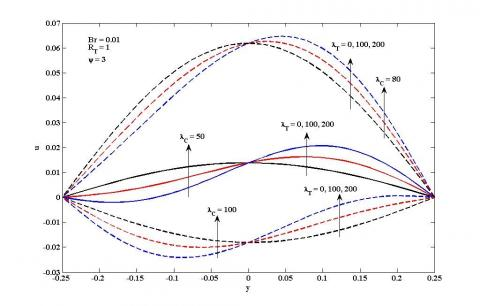Figure 2. Velocity profile for different values of $\lambda_{C}$ and $\lambda_{T}$ when $a=1$

## f3.jpgFigure 3. Velocity profile for different values of $\lambda_{C}$ and $a$

## f4.jpg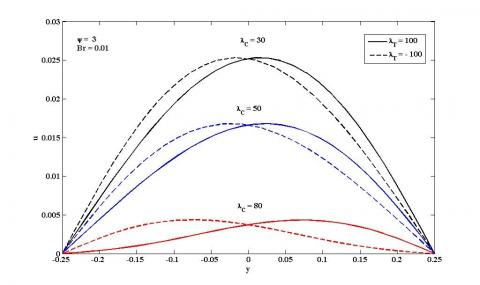Figure 4. Velocity profile for different values of $\lambda_{C}$ for $\lambda_{T}=\pm 100$ when $\mathrm{a}=1$

Figure 5 shows the effect of heat generation/absorption parameter ѱ. Temperature profile is linear for lower values of ѱ and nonlinear for higher values. Also there is an increase in the profile for higher values of ѱ. The increase is due to increase in internal heat.

In the Figure 6 the effect of α is shown. It is evident that increased values of α enhances the concentration which results in the increased profile near the left wall of higher concentration till the middle of the channel and symmetrically decreased profile near the lower concentration right wall. This is because the diffusion is faster near the higher concentration region.

## f5.jpg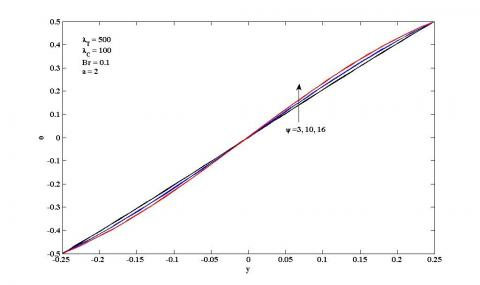Figure 5. Temperature profile for different values of ѱ

## f6.jpg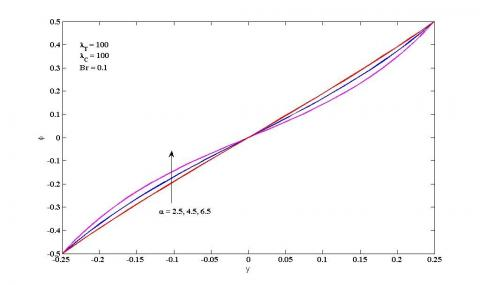Figure 6. Concentration ϕvs y for different values of α

Figure 7 and 8 presents the velocity and temperature profiles for isoflux – isothermal boundary conditions. The profiles are obtained for $\lambda_{T}=\pm 100$. For the positive value of $\lambda_{T}$ velocity increases, also decreases for negative value of $\lambda_{T}$. The temperature profile, for constant heat flux on the left wall, temperature increases for both positive and negative values of $\lambda_{T}$ for $t=1,1.5,2$.

Figure 9 and 10 illustrate the velocity and temperature profiles for isothermal – isoflux boundary conditions. In this case also the profiles are obtained for $\lambda_{T}=\pm 500$. For the positive value of $\lambda_{T}$ velocity decreases, also increases for negative value of $\lambda_{T}$. The temperature profile increases for both positive and negative values of $\lambda_{T}$ or $\lambda_{T}$ for $t=1,1.5,2$

Table 1 and 2 reveal that the Nusselt number increases on the left wall and decreases on the right wall for $\lambda_{T}=100, \lambda_{T}=-100$ for different values of couple stress parameter $a=4$ and $a=6$. Table 3 and 4 explains the skin friction on both the walls, which shows increase on the left and decrease on the right wall. Table 5 shows Sherwood number for different values of αrevealing that concentration transfer increases on both left and right walls uniformly because, the diffusion of concentration is uniform.

## f7.jpg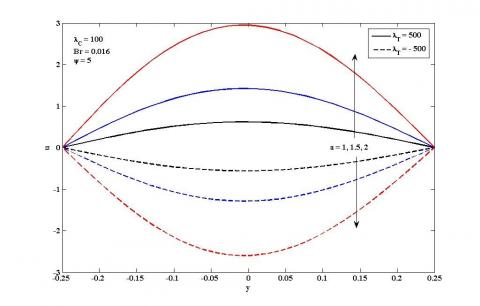Figure 7. Velocity uvs y for different values $a$ of in the case of isoflux – isothermal

## f8.jpg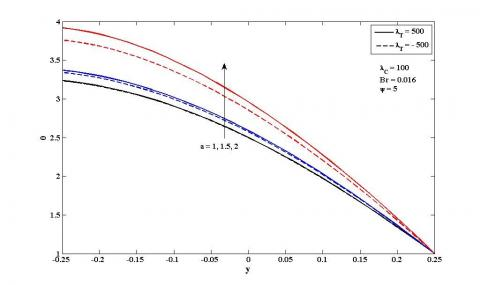Figure 8. Temperature θvs y for different values a of in the case of isoflux – isothermal

## f9.jpgFigure 9. Velocity u vs y for different values a of in the case of isothermal – isoflux

## f10.jpg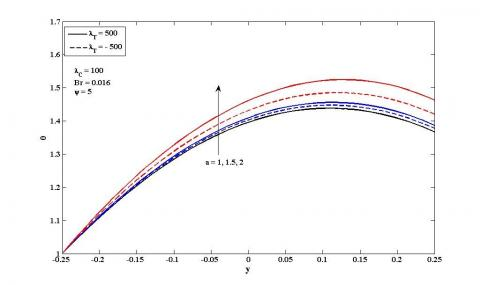Figure 10. Temperature θvs y for different

Table 1. Nusselt number for different values of a

 $\lambda_{T}=100$ $\varepsilon=\lambda_{T} B r$ $a=4$ $a=6$ $\mathrm{N} u_{1}$ $\mathrm{N} u_{2}$ $\mathrm{N} u_{1}$ $\mathrm{N} u_{2}$ 0 1.7872 1.7872 1.7872 1.7872 0.5 1.7875 1.7870 1.7881 1.7867 1 1.7878 1.7869 1.7891 1.7863 2 1.7884 1.7865 1.7909 1.7854 3 1.7890 1.7862 1.7928 1.7845 4 1.7897 1.7859 1.7946 1.7836 5 1.7903 1.7856 1.7965 1.7827

Table 2. Nusselt number for different values of a

 $\lambda_{T}=-100$ $\varepsilon=\lambda_{T} B r$ $a=4$ $a=6$ $\mathrm{N} u_{1}$ $\mathrm{N} u_{2}$ $\mathrm{N} u_{1}$ $\mathrm{N} u_{2}$ 0 1.7872 1.7872 1.7872 1.7872 0.5 1.7874 1.7869 1.7876 1.7862 1 1.7875 1.7866 1.7881 1.7852 2 1.7878 1.7859 1.7890 1.7833 3 1.7882 1.7853 1.7899 1.7813 4 1.7885 1.7846 1.7909 1.7793 5 1.7888 1.7840 1.7918 1.7774

Table 3. Skin friction for different values of a

 $\lambda_{T}=500$ $\varepsilon=\lambda_{T} B r$ $a=4$ $a=6$ $\tau_{1}$ $\tau_{2}$ $\tau_{1}$ $\tau_{2}$ 0 -1.8430 -0.7556 -3.5408 -1.7394 0.5 -1.8424 -0.7562 -3.5368 -1.7434 1 -1.8417 -0.7568 -3.5327 -1.7473 2 -1.8405 -0.7580 -3.5248 -1.7551 3 -1.8392 -0.7593 -3.5168 -1.7630 4 -1.8380 -0.7605 -3.5089 -1.7708 5 -1.8368 -0.7617 -3.5010 -1.7785

Table 4. Skin friction for different values of a

 $\lambda_{T}=500$ $\varepsilon=\lambda_{T} B r$ $a=4$ $a=6$ $\tau_{1}$ $\tau_{2}$ $\tau_{1}$ $\tau_{2}$ 0 0.7719 1.8592 1.7723 3.5736 0.5 0.7712 1.8599 1.7683 3.5777 1 0.7706 1.8605 1.7642 3.5819 2 0.7693 1.8618 1.7561 3.5901 3 0.7681 1.8631 1.7480 3.5984 4 0.7668 1.8644 1.7398 3.6068 5 0.7656 1.8657 1.7316 3.6151

Table 5. Sherwood number for different values of α

 $\alpha$ $S h_{1}$ $S h_{2}$ 0 2.0000 2.0000 0.5 2.0104 2.0104 1.5 2.0929 2.0929 2 2.1640 2.1640 2.5 2.2539 2.2539
5. Conclusion

The effect of viscous dissipation, heat generation/absorption and first order chemical reaction on laminar combined free and forced convection through vertical channel with couple stress fluid has been studied numerically with isothermal – isothermal, isoflux – isothermal, isothermal – isoflux boundary conditions are considered for walls. The non – dimensional coupled nonlinear equations have been solved numerically. The Nusselt number for heat transfer and Sherwood number for concentration have been evaluated and represented by tables. It is evident from this study that effect of viscous dissipation, heat generation/absorption and first order chemical reaction is dominant in case of upward flow. The presence of viscous dissipation increases the flow regime. Prime importance of the study is the effect of chemical reaction, which enhances the velocity, temperature. In the case of isoflux – isothermal, isothermal – isoflux boundary conditions the velocity increases for positive value and decreases for negative values of heat generation/absorption parameter. Temperature also increases in both the conditions. Nusselt numbers show that heat transfer at left wall increases and decreases at the right values.

Nomenclature
 C1, C2 boundary concentrations [K] C0 reference concentration L channel width [m] g acceleration due to gravity [m s-2] GrC concentration Grashoff constant GrT thermal Grashoff constant H = 2L hydraulic diameter [m] K thermal conductivity Nu1, Nu2 Nusselt numbers defined in (24) p pressure [Pa] RC concentration difference ratio Re Reynolds number RT temperature difference ratio concentration u dimensionless velocity component along X – axis Sh1 ,Sh2 Sherwood numbers as in (25 T temperatures [K] T1, T2 boundary temperatures [K] T0 reference temperature U velocity component along X – axis [m s-1] U0 reference velocity X streamwise coordinate[m] y dimensionless transverse coordinate Y transverse coordinate [m] D concentration diffusivity constant

Greek letters

 α concentration arameter α1 thermal diffusivity θ dimensionless temperature Φ dimensionless concentration μ dynamic viscosity [Pa s] βT thermal expansion coefficient φ heat generation/absorption parameter ν kinematic viscosity [m s-1] η couplestress  parameter βC concentrationexpansion coefficient
References

 Umavathi JC, Prathap Kumar J, Chamkha J. (2009). Convective flow of two immiscible viscous and couple stres permeable fluids through a vertical channel. Tuskish J. Eng. Env. Sci. 33: 221–243. https://doi.org/10.3906/muh-0905-29

 Malashetty MS, Umavathi JC. (1999). Oberbeck convective flow of a couple stress fluid through a vertical porous stratum. Int. J. Non-Linear Mech 34: 1037-1045. https://doi.org/10.1016/S00207462(98)00074-2

 Sinha P, Singh C. (1999). Effects of couple stress on the blood flow through and artery with mild stenosis. Biorheology 21: 303-312.

 Umavathi JC. (1999). Free convection flow of electrically conducting couple stress fluid in a vertical channel. ASME 68: 1-24. https://doi.org/10.1108/HFF-12-2015-0540

 Umavathi JC, Manjula MH, Liu IC, Pop I. (2007). Flow and heat transfer of couple stress and viscous fluids in a vertical channel. In. J. Appl. And Engng 12: 537–555.

 Valanis KC, Sun CT. (1969). Poiseuille flow of a fluid with a couple stress with applications to blood flow. Biorheology 6: 85–97. https://doi.org/10.3233/BIR-1969-6203

 Zanchini E. (1998). Effect of viscous dissipation on mixed convection in a vertical channel with boundary conditions of the third kind. International Journal of Heat and Mass Transfer 3949-3959. https://doi.org/10.1016/S0017-9310(98)00114-8

 Barletta A. (1998). Laminar mixed convection with viscous dissipation in a vertical channel. Internal Journal of Heat and Mass Transfer 41: 3501-3513. https://doi.org/10.1016/S0017-9310(98)00074-X

 Berletta A, Zanchini E. (1999). On the choice of the reference temperature for fully developed mixed convection in a vertical channel. International Journal of Heat and Mass Transfer 42: 3169-3181. https://doi.org/10.1016/S0017-9310(99)00011-3

 Berletta A. (1999). Heat transfer by fully developed flow and viscous heating in a vertical channel with prescribed wall heat fluxes. International Journal of Heat and Mass Transfer 42(20): 3873-3885. doi:10.1016/S0017-9310(99)00060-5

 Anjalidevi SP, Kandansamy R. (1999). Effects of chemical reaction, heat and mass transfer on laminar flow along a semi-infinite horizontal plate. Heat Mass Transfer 35: 465-467. https://doi.org/10.1007/s002310050349

 Prathap Kumar J, Umavathi JC, Sharadkumar J. (2013). Effect of first order chemical reaction in a vertical double-passage channel. Int. Journal of Engineering Research and Application 3(5): 967-977.

 Prathap Kumar J, Umavathi JC, Sharadkumar J. (2014). Effect of first order chemical reaction in a vertical double passage channel filled with porous matrix. International Journal of Mathematical Archive 5(12): 94-116.

 Muthucumaraswamy R, Ganesan P. (2001). Effect of the chemical reaction and injection on flow characteristics in an unsteady upward motion of an isothermal plate. J. Appl. Mech Tech Phys 42: 665-671. https://doi.org/10.1023/A:1019259932039

 Seddeek MK. (2005). Finite element method for the effects of chemical reaction, variable viscosity, thermophoresis and heat generation/absorption on a boundary layer hydromagnetic flow with heat and mass transfer over a heat surface. ActaMech 177: 1-18. https://doi.org/10.1007/s00707-005-0249-8

 Prathap Kumar J, Umavathi JC, Shivakumar M. (2012). Effect of homogeneous and heterogeneous reaction on solute dispersion in composite porous medium. Int. J. Eng. Sci. Techno. 4: 58-76.

 Muthucumaraswamy R, Ganesan P. (2001). First order chemical reaction on flow past an impulsively started vertival plate with uniform heat and mass flux. Acta Mechanica 147: 45-57. https://doi.org/10.1007/BF01182351

 Prasad KV, Mallikarjun P, Vaidya H. (2017). Mixed convective fully developed flow in a vertical channel in the presence of thermal radiation and viscous dissipation. Int. J. of Applied Mechanics and Engineering 22(1): 123-144. https://doi.org/10.1515/ijame-2017-0008

 Umavathi JC, PatilMallikarjun B, Narasimha Murthy S. (2013). On laminar magneto convection flow in a Vertical channel in the presence of heat generation and absorption. Journal of Heat Transfer 135: 1-9. https://doi.org/10.1115/1.4023222